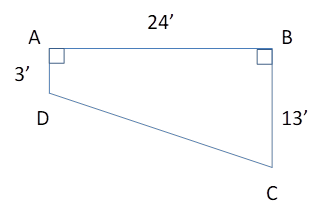Chapter 4.4, Problem 41EElementary Geometry For College St...

7th Edition
Alexander + 2 others
ISBN: 9781337614085

Solutions

Chapter
SectionElementary Geometry For College St...

7th Edition
Alexander + 2 others
ISBN: 9781337614085
Textbook Problem

The vertical side wall of an in-ground pool that is 24 ft in length has the shape of a right trapezoid. What is the depth of the pool in the middle?To determine

To Find:

The depth of the pool in the middle.

Explanation

Consider the following diagram.

The length of the vertical side wall is 24 ft.

The pool has the shape of a right trapezoid and the lengths of two bases are 3 ft. and 13 ft.

The depth is low at the point D and it increases from D to C.

We need to find the depth of the pool in the middle.

The length of the median gives the depth of the pool in the middle...

Still sussing out bartleby?

Check out a sample textbook solution.

See a sample solution

The Solution to Your Study Problems

Bartleby provides explanations to thousands of textbook problems written by our experts, many with advanced degrees!

Get Started

True or False: If and , then .

Study Guide for Stewart's Multivariable Calculus, 8th

In the graph at the right, limx1f(x)=. a) 2 b) 1 c) 3 d) does not exist

Study Guide for Stewart's Single Variable Calculus: Early Transcendentals, 8th

In Exercises 21-26, use the laws of logic to prove the propositions. (pq)qt

Finite Mathematics for the Managerial, Life, and Social Sciences